# Tangent + polygon - math problems

Tangent is a trigonometric function. In a rectangular triangle, it is the ratio of the opposite and adjacent side to a given internal angle. Algebraically is defined as the ratio of the sine and cosine of a given angle. It is periodic with a period of π = 180 °.

#### Number of problems found: 14

• Find the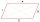Find the content of a regular 12 sided polygon, if its side a = 12 cm.Calculate the content of a regular 15-sides polygon inscribed in a circle with radius r = 4. Express the result to two decimal places.
• Regular n-gonWhich regular polygon have a radius of circumscribed circle r = 10 cm and the radius of inscribed circle p = 9.962 cm?
• Inscribed and described circle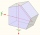Find the radii of a circle inscribed and circumscribed by a regular pentagon whose side measures 3 cm.
• n-gon IIWhat is the side length of the regular 5-gon circumscribed circle of radius 11 cm?
• Regular 5-gonCalculate the area of the regular pentagon with side 7 cm.
• Pentagonal pyramid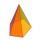Calculate the volume of a regular 5-side (pentaprism) pyramid ABCDEV; if |AB| = 7.7 cm and a plane ABV, ABC has angle 37 degrees.
• Diagonals of pentagonCalculate the diagonal length of the regular pentagon: a) inscribed in a circle of radius 12dm; b) a circumscribed circle with a radius of 12dm.
• Octagonal pyramidFind the volume of a regular octagonal pyramid with height v = 100 and the angle of the side edge with the plane of the base is α = 60°.
• Decagon prism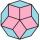A regular decagon of side a = 2 cm is the base of the perpendicular prism, the side walls are squares. Find the prism volume in cm3, round to two decimal places.
• 4side pyramidCalculate the volume and surface of 4 sides regular pyramid whose base edge is 4 cm long. The angle from the plane of the sidewall and base plane is 60 degrees.
• Which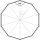Which of the following numbers most accurately area of a regular decagon with side s = 2 cm? (A) 9.51 cm2 (B) 20 cm2 (C) 30.78 cm2 (D) 31.84 cm2 (E) 32.90 cm2
• GlassHow many glass are needed to produce glass with base regular 5-gon if one base triangle in the base is 4.2 square cm and the height is 10 cm?
• NonagonCalculate the area and perimeter of a regular nonagon if its radius of the inscribed circle is r = 10cm

We apologize, but in this category are not a lot of examples.
Do you have an interesting mathematical word problem that you can't solve it? Submit a math problem, and we can try to solve it.

We will send a solution to your e-mail address. Solved examples are also published here. Please enter the e-mail correctly and check whether you don't have a full mailbox.

Please do not submit problems from current active competitions such as Mathematical Olympiad, correspondence seminars etc...